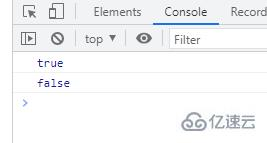# es6如何判断两个字符串是否相等

es6判断两个字符串是否相等

`==`运算符，可以比较等式两边的值是否相等

```var x = "hello";
var y = "HELLO";
var z = "hello";
console.log(x == y);  // 输出： false
console.log(x == z);  // 输出： true```Object.is() 方法判断两个值是否为同一个值。如果满足以下条件则两个值相等：

• 都是 undefined

• 都是 null

• 都是 true 或 false

• 都是相同长度的字符串且相同字符按相同顺序排列

• 都是相同对象（意味着每个对象有同一个引用）

• 都是数字且

• 都是 +0

• 都是 -0

• 都是 NaN

• 或都是非零而且非 NaN 且为同一个值

```var x = "hello";
var y = "HELLO";
var z = "hello";
console.log(Object.is(x,z));  // 输出： true
console.log(Object.is(x,y));  // 输出： false```